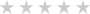Reviews
Target Audience
Course Overview
Course Requirements
Course Syllabus
-

This module explains how to work with advanced formulas in Microsoft Excel.

Lessons

• Using Named Ranges in Formulas
• Using Named Ranges in Formulas
• Using Formulas That Span Multiple Worksheets
• Entering a Formula Using Data in Multiple Worksheets
• Using the IF Function
• Using the IF Function
• Using the PMT Function
• Using the PMT Function
• Using the LOOKUP Function
• Using the VLOOKUP Function
• Using the VLOOKUP Function
• Using the HLOOKUP Function
• Using the CONCAT Function
• Using the CONCAT Function
• Using the TRANSPOSE Function
• Using the PROPER, UPPER, and LOWER Functions
• Using the PROPER Function
• Using the LEFT, RIGHT, and MID Functions
• Using the LEFT and RIGHT Functions
• Using Date Functions
• Using the YEAR, MONTH, and DAY Functions
• Creating Scenarios

• Using Named Ranges in Formulas
• Entering a Formula Using Data in Multiple Worksheets
• Using the IF Function
• Using the PMT Function
• Using the VLOOKUP Function
• Using the CONCAT Function
• Using the PROPER Function
• Using the LEFT and RIGHT Functions
• Using the YEAR, MONTH, and DAY Functions
After completing this module, students will be able to:
• Name and label cells and ranges of cells.
• Use names and labels in formulas.
• Create formulas that span multiple worksheets.
• Use the conditional IF function and its variants in formulas.
• Use the PMT function to calculate payments for loans.
• Use the LOOKUP function.
• Use the VLOOKUP function.
• Use the HLOOKUP function.
• Use the CONCAT function to join the contents of numerous cells.
• Use the TRANSPOSE function.
• Use the PROPER, UPPER, and LOWER functions to alter the casing of text.
• Use the LEFT, RIGHT, and MID functions to return characters from the
• start or end of a string, or a specific number of text characters.
• Use various date functions.
-

Lessons

• Converting a List to a Table
• Converting a List to a Table
• Removing Duplicates from a List
• Removing Duplicates from a List
• Sorting Data in a List
• Sorting Data in a List
• Filtering Data in a List
• Filtering Data in a List
• Adding Subtotals to a List

Lab 1: Work with Lists Exercises

• Converting a List to a Table
• Removing Duplicates from a List
• Sorting Data in a List
• Filtering Data in a List
• Adding Subtotals to a List
After completing this module, students will be able to:
• Convert data into tables.
• Remove duplicates from tables.
• Sort data in Excel.
• Filter data in Excel.
• Use subtotals to automatically total related data.
• Group and ungroup data.
-

Module 3: Working with Illustrations

This module explains how to work with Illustrations in Microsoft Excel.

Lessons

• Working with Clip Art
• Working with Clip Art
• Using Shapes
• Working with Icons
• Working with SmartArt
• Using Office Ink

Lab 1: Working with Illustrations Exercises

• Working with Clip Art
After completing this module, students will be able to:
• Use Clip Art to illustrate your worksheets.
• Insert shapes into Microsoft Excel worksheets.
• Use SmartArt to illustrate your worksheets.
-

This module explains how to work with various types of charts in Microsoft Excel.

Lessons

• Inserting Charts
• Using the Chart Recommendation Feature
• Inserting Charts
• Editing Charts
• Using Chart Tools
• Using the Quick Analysis Tool
• Editing Charts
• Create a Custom Chart Template

Lab 1: Visualizing Your Data Exercises

• Inserting Charts
• Editing Charts
After completing this module, students will be able to:
• Create charts that enable you to visualize your data.
• Choose what data is displayed in your charts.
• Show and hide data labels.
• Show and hide the legend.
• Show and hide the chart title.
• Add a picture or shape to a chart.
• Change the way text displays in a chart.
• Change the fill color of a chart.
• Add and format objects in a worksheet.
• Create a custom chart template.
-

Module 5: Working with Tables

This module explains how to work with tables in Microsoft Excel.

Lessons

• Format Data as a Table
• Move between Tables and Ranges
• Modify Tables
• Define Titles
• Creating and Modifying a Table in Excel

Lab 1: Creating and Modifying a Table in Excel

After completing this module, students will be able to:
• Format data in Excel as a table.
• Modify Excel tables
-

This module explains how to work with advanced formatting features in Microsoft Excel.

Lessons

• Applying Conditional Formatting
• Using Conditional Formatting
• Working with Styles
• Working with Styles
• Creating and Modifying Templates